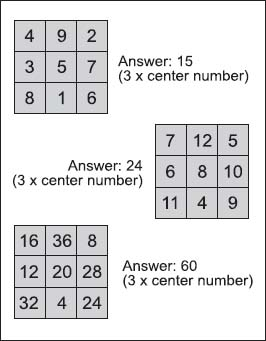NextPrevious

# What is a magic square?In these 3-by-3 Chinese magic squares, the numbers are arranged so that when added vertically, horizontally, or diagonally, they are always equal to 3 times the center number.

A magic square is an array of numbers in an n by n square that contains positive integers—from 1 to n2—with each number occurring only once. The numbers in the squares are arranged so that the sum in any horizontal, vertical, or main diagonal direction is always the same. This is shown in the following formula: n(n2 + 1)/2.

Magic squares are often divided into orders; for example, a three-order magic square means three boxes per row and three boxes per column. In reality, “magic” squares are actually matrices (for more information about a matrix, see “Algebra”); they can be odd-order (such as a 3-by-3, or 5-by-5 matrix) or even-order (such as a 4-by-4 or 6-by-6 matrix) magic squares. Perhaps the simplest magic square is the 1-by-1 square, with the only entry being the number 1.

Such magic squares have been known for centuries. For example, the Chinese knew about the three unique normal squares of order three. In Chinese literature dating from as early as 2800 B.C.E., a magic square known as the Loh-Shu, or “scroll of the river Loh,” was invented by Fuh-Hi, who is thought of as the mythical founder of the Chinese civilization.

Close

This is a web preview of the "The Handy Math Answer Book" app. Many features only work on your mobile device. If you like what you see, we hope you will consider buying. Get the App